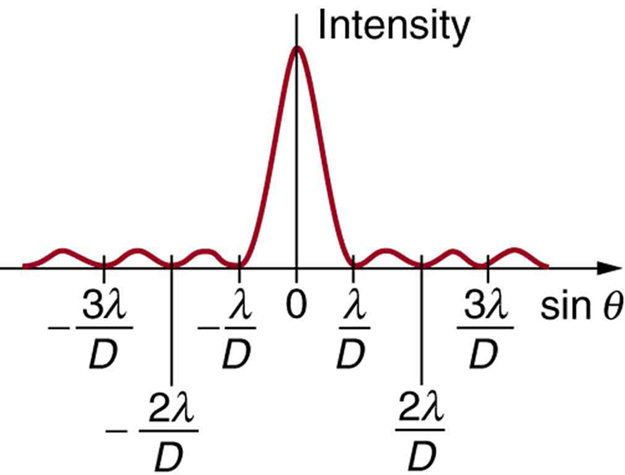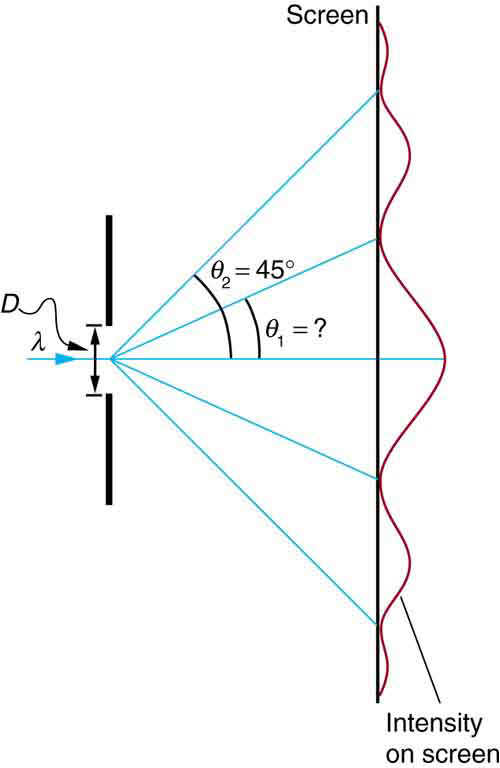# 27.5 Single slit diffraction  (Page 2/4)

 Page 2 / 4A graph of single slit diffraction intensity showing the central maximum to be wider and much more intense than those to the sides. In fact the central maximum is six times higher than shown here.

Thus, to obtain destructive interference for a single slit    ,

$D\text{sin}\phantom{\rule{0.25em}{0ex}}\theta =\mathrm{m\lambda },\phantom{\rule{0.25em}{0ex}}\text{for}\phantom{\rule{0.25em}{0ex}}m=\text{1,}\phantom{\rule{0.25em}{0ex}}\text{–1,}\phantom{\rule{0.25em}{0ex}}\text{2,}\phantom{\rule{0.25em}{0ex}}\text{–2,}\phantom{\rule{0.25em}{0ex}}\text{3,}\phantom{\rule{0.25em}{0ex}}\dots \phantom{\rule{0.25em}{0ex}}\text{(destructive),}$

where $D$ is the slit width, $\lambda$ is the light’s wavelength, $\theta$ is the angle relative to the original direction of the light, and $m$ is the order of the minimum. [link] shows a graph of intensity for single slit interference, and it is apparent that the maxima on either side of the central maximum are much less intense and not as wide. This is consistent with the illustration in [link] (b).

## Calculating single slit diffraction

Visible light of wavelength 550 nm falls on a single slit and produces its second diffraction minimum at an angle of $\text{45.0º}$ relative to the incident direction of the light. (a) What is the width of the slit? (b) At what angle is the first minimum produced?A graph of the single slit diffraction pattern is analyzed in this example.

Strategy

From the given information, and assuming the screen is far away from the slit, we can use the equation $D\text{sin}\phantom{\rule{0.25em}{0ex}}\theta =\text{mλ}$ first to find $D$ , and again to find the angle for the first minimum ${\theta }_{1}$ .

Solution for (a)

We are given that $\lambda =\text{550 nm}$ , $m=2$ , and ${\theta }_{2}=\text{45.0º}$ . Solving the equation $D\phantom{\rule{0.25em}{0ex}}\text{sin}\phantom{\rule{0.25em}{0ex}}\theta =\text{mλ}$ for $D$ and substituting known values gives

$\begin{array}{lll}D& =& \frac{\mathrm{m\lambda }}{\phantom{\rule{0.25em}{0ex}}\text{sin}\phantom{\rule{0.25em}{0ex}}{\theta }_{2}}=\frac{2\left(\text{550 nm}\right)}{\text{sin 45.0º}}\\ & =& \frac{\text{1100}×{\text{10}}^{-9}}{0.707}\\ & =& 1.56×{\text{10}}^{-6}.\end{array}$

Solution for (b)

Solving the equation $D\phantom{\rule{0.25em}{0ex}}\text{sin}\phantom{\rule{0.25em}{0ex}}\theta =\text{mλ}$ for $\text{sin}\phantom{\rule{0.25em}{0ex}}{\theta }_{1}$ and substituting the known values gives

$\text{sin}\phantom{\rule{0.25em}{0ex}}{\theta }_{1}=\frac{\mathrm{m\lambda }}{D}=\frac{1\left(\text{550}×{\text{10}}^{-9}\phantom{\rule{0.25em}{0ex}}\text{m}\right)}{1\text{.}\text{56}×{\text{10}}^{-6}\phantom{\rule{0.25em}{0ex}}\text{m}}\text{.}$

Thus the angle ${\theta }_{1}$ is

${\theta }_{1}={\text{sin}}^{-1}\phantom{\rule{0.25em}{0ex}}\text{0.354}=\text{20.7º.}$

Discussion

We see that the slit is narrow (it is only a few times greater than the wavelength of light). This is consistent with the fact that light must interact with an object comparable in size to its wavelength in order to exhibit significant wave effects such as this single slit diffraction pattern. We also see that the central maximum extends $\text{20.7º}$ on either side of the original beam, for a width of about $\text{41º}\phantom{\rule{0.25em}{0ex}}$ . The angle between the first and second minima is only about $\text{24º}\phantom{\rule{0.25em}{0ex}}\left(\text{45.0º}-20.7º\right)$ . Thus the second maximum is only about half as wide as the central maximum.

## Section summary

• A single slit produces an interference pattern characterized by a broad central maximum with narrower and dimmer maxima to the sides.
• There is destructive interference for a single slit when $D\phantom{\rule{0.25em}{0ex}}\text{sin}\phantom{\rule{0.25em}{0ex}}\theta =\text{mλ},\phantom{\rule{0.25em}{0ex}}\text{(for}\phantom{\rule{0.25em}{0ex}}m=\text{1,}\phantom{\rule{0.25em}{0ex}}\text{–1,}\phantom{\rule{0.25em}{0ex}}\text{2,}\phantom{\rule{0.25em}{0ex}}\text{–2,}\phantom{\rule{0.25em}{0ex}}\text{3,}\phantom{\rule{0.25em}{0ex}}\dots \right)$ , where $D$ is the slit width, $\lambda$ is the light’s wavelength, $\theta$ is the angle relative to the original direction of the light, and $m$ is the order of the minimum. Note that there is no $m=0$ minimum.

## Conceptual questions

As the width of the slit producing a single-slit diffraction pattern is reduced, how will the diffraction pattern produced change?

## Problems&Exercises

(a) At what angle is the first minimum for 550-nm light falling on a single slit of width $1\text{.}\text{00}\phantom{\rule{0.25em}{0ex}}\text{μm}$ ? (b) Will there be a second minimum?

(a) $\text{33}\text{.}4º$

(b) No

(a) Calculate the angle at which a $2\text{.}\text{00}\text{-μm}$ -wide slit produces its first minimum for 410-nm violet light. (b) Where is the first minimum for 700-nm red light?

What is the difference between a principle and a law
the law is universally proved. The principal depends on certain conditions.
Dr
what does the speedometer of a car measure ?
Car speedometer measures the rate of change of distance per unit time.
Moses
describe how a Michelson interferometer can be used to measure the index of refraction of a gas (including air)
using the law of reflection explain how powder takes the shine off a person's nose. what is the name of the optical effect?
WILLIAM
is higher resolution of microscope using red or blue light?.explain
WILLIAM
can sound wave in air be polarized?
Unlike transverse waves such as electromagnetic waves, longitudinal waves such as sound waves cannot be polarized. ... Since sound waves vibrate along their direction of propagation, they cannot be polarized
Astronomy
A proton moves at 7.50×107m/s perpendicular to a magnetic field. The field causes the proton to travel in a circular path of radius 0.800 m. What is the field strength?
derived dimenionsal formula
what is the difference between mass and weight
assume that a boy was born when his father was eighteen years.if the boy is thirteen years old now, how is his father in
Isru
what is airflow
derivative of first differential equation
why static friction is greater than Kinetic friction
draw magnetic field pattern for two wire carrying current in the same direction
An American traveler in New Zealand carries a transformer to convert New Zealand’s standard 240 V to 120 V so that she can use some small appliances on her trip.
What is the ratio of turns in the primary and secondary coils of her transformer?
nkombo
what is energy
Yusuf
How electric lines and equipotential surface are mutually perpendicular?
The potential difference between any two points on the surface is zero that implies È.Ŕ=0, Where R is the distance between two different points &E= Electric field intensity. From which we have cos þ =0, where þ is the angle between the directions of field and distance line, as E andR are zero. Thus
sorry..E and R are non zero...

#### Get Jobilize Job Search Mobile App in your pocket Now!ByBy Brooke DelaneyBy Madison ChristianBy OpenStaxByBy Sheila LopezBy Angela EckmanBy Cameron CaseyBy Richley CrapoBy OpenStaxBy OpenStax